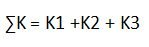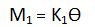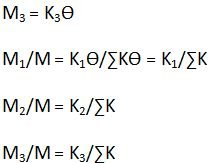# Moment Distribution Method | Civil Engineering

#### Step One:

Initially all the members are assumed to be fixed. Calculation of fixed end moments is to be done.

#### Step two:

Fixidity at the joint is released and the balancing moment at the joints is distributed to various members depending upon their thickness.

#### Step three:

A part of this moment is carried over to the other end of the member and the carry over moment is computed.

#### Step four:

This process of distribution and carry over is continued till the unbalanced moment at the joints become zero or negligible.

#### Carry Over factor

COF = Moment induced at one end/Moment applied at the other end

One end hinged and the other end fixed, COF = ½

For both ends hinged, COF = 0

#### Stiffness (k)

Fixed:

K = Moment of inertia/length of the member = I/L

For Hinged:

K = 3I/4L

Now, we shall discuss “Distribution Factor” which is very important while carrying out calculations using Moment Distribution Method.

#### Distribution Factor

Have a look at the diagram below.

For the member OA with end A fixed,

K1 = 4EI1/L1

For the member OB with end B fixed,

K2 = 4EI2/L2

For the member OC with end C hinged,

K3 = 3EI3/L3

Total stiffness of the member is given by,And the total moment applied at the joint ‘O’Moment on the member OA is given by,Moment on the member OB is given by,Moment on the member OC is given by,are the distribution factors for OA, OB and OC respectively.

M1, M2 and M3 are the distribution moments.

With this, we come to the end of our step by step procedure for “Moment Distribution method”. We would also be studying MDM with the help of examples in our succeeding articles.

### 4 thoughts on “Moment Distribution Method | Civil Engineering”

1.I want a calculation for the continuity bending moments of a beam….one end is fixed marked “A”……The beam extends (UDL = 6 kN/m) and supported by a roller at 8 m from “A”…marked “B”…..then overhang 2 meters from “B” (Not UDL) but a point load applied at end of overhang of magnitude 10 kN….Can you calculate the positive bending moment at midspan of beam AB….and sketch the final bending moment diagram….also compute the beam reaction at roller support “B”….

2.please notify me of other methods of analyzing structures, more so simple analysis of multi-storied structures

3.4.